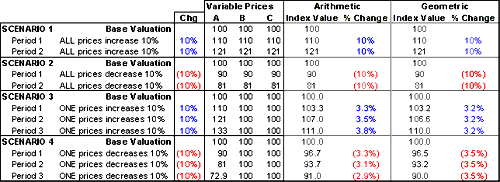# Riding The Wave

September 01, 2005

Arithmetic Vs. Geometric-An Example

In practice, geometric indexes will always underperform arithmetic indexes-geometrically averaged indexes rise slower and fall faster than arithmetically averaged indexes. Figure 7 helps to highlight the differences between arithmetic and geometric averaging methods. Consider an index comprised of only three components-A, B and C-and assume that these components are equally weighted. The base period price for each scenario is assumed to be 100.

Figure 7The data make clear several important points:

•  Equal price changes in all components will result in equal index changes for both geometrically and arithmetically averaged indexes (scenarios 1 & 2).

•  The impact from equal, successive percentage changes in one component are constant for geometric averages. Thus, a 10 percent increase in an individual commodity, all others held constant, will always cause a geometric index to increase by 3.2 percent. Conversely, a 10 percent decrease in an individual commodity, all others held constant, will cause the geometric index to decrease by 3.5 percent (scenarios 3 & 4).4

•  When component prices move independently, geometrically averaged indexes rise slower and fall faster than arithmetically averaged indexes. Scenario 3 shows the geometric index value rising at a constant 3.2 percent while the arithmetic index changes at an accelerating rate (from 3.3 to 3.8 percent). Scenario 4 shows the geometric index value falling by a constant rate (again, 3.2 percent) while the arithmetic index falls at a decelerating rate (from 3.3 to 2.9 percent). This has significant implications for hedgers and others wishing to manage exposure to geometrically indexed products.

•  For an arithmetic index, successive constant percentage price changes in one component increase with rising prices and decrease with falling prices (scenarios 3 & 4).

•  In geometric averaging, changes in an individual component's price do not change the component's relative dollar weight in the index.

• In arithmetic averaging, the relative weight of an individual component will change as the component prices move independently of each other. Scenario 3 illustrates that as the price of component A increases at a constant rate of 10 percent each period (all other prices held constant), the index value increases at a rate that accelerates from 3.3 to 3.8 percent (i.e., the component is more heavily weighted). Conversely, as the price of component A declines at a constant rate of 10 percent (scenario 4), the impact on the index price level decreases (from 3.3 percent to 2.9 percent).

• A last point highlights a dynamic that has important ramifications for investors and should be discussed in more detail. In arithmetic averages, component concentrations within the index are subject to the relative price changes of the components. When the price of a single component rises relative to other index constituents, the weight of that component within the index will also rise. Similarly, when a constituent price falls relative to the others, its weight within the index will decrease. As an example, look at the GSCI for the period from January of this year until the beginning of July. The Energy sector represented just under 67 percent of the index valuation at the start of the period. By the end, this level of exposure increased to almost 77 percent, re p resenting a rise in the sector weight of more than ten percent. For an investor looking to gain exposure to raw energy products, this was a good position to take. It may not have been as suitable if the aim was to gain broad exposure to a diversified commodity basket.